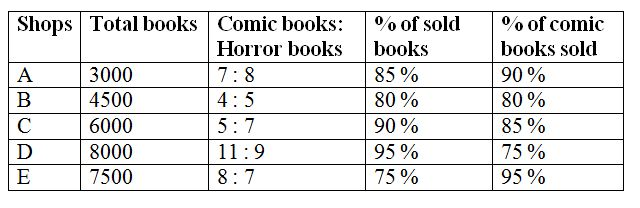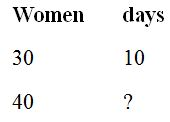# SBI Clerk/ IDBI and South Indian Bank Mains Quantitative Aptitude Questions 2019 (Day-02)

Dear Aspirants, Our IBPS Guide team is providing a new series of Quantitative Aptitude Questions for SBI Clerk /IDBI and South Indian Bank Mains 2019 so the aspirants can practice it on a daily basis. These questions are framed by our skilled experts after understanding your needs thoroughly. Aspirants can practice these new series questions daily to familiarize with the exact exam pattern and make your preparation effective.

Check here for IBPS RRB Clerk Prelims Mock Test 2019

Check here for EPFO Assistant Mock Test 2019

Check here for LIC ADO Mock Test 2019

[WpProQuiz 6671]

Directions (Q. 1 – 5): Study the following information carefully and answer the given questions.

The following table shows the total number of books (Comic and Horror) in 5 different shops and the ratio of comic to that horror books among them and the percentage of books sold by those shops in a certain year.1) Find the difference between the total number of horror books sold by shop A to that of total number of horror books sold by shop D?

a) 3010

b) 2950

c) 2740

d) 2560

e) None of these

2) Find the ratio between the total number of comic books in shop A, B and C together to that of total number of horror books in shop C, D and E together?

a) 73 : 147

b) 32 : 85

c) 95 : 173

d) 59 : 106

e) None of these

3) Find the average number of books sold by all the given shops together?

a) 4270

b) 4955

c) 4785

d) 4520

e) None of these

4)  Find the ratio between the percentage of horror books sold by shop B out of the total books in shop B to that of shop C?

a) 75 : 83

b) 129 : 137

c) 320 : 393

d) 157 : 216

e) None of these

5) Total number of comic books sold by shop A is approximately what percentage more/less than the total number of horror books sold by shop E?

a) 31 % more

b) 12 % less

c) 12 % more

d) 45 % more

e) 31 % less

6) 8 men and 10 women can complete a piece of work in 10 days while 6 men and 10 women can complete the same work in 12 days. Then find the number of days taken by 10 men and 15 women to complete the same work?

a) 7 ½ days

b) 9 days

c) 10 ½ days

d) 11 ¾ days

e) None of these

7) A circular path is surrounding the circular plot is being gravelled at a total cost of Rs. 5390 at Rs. 5 per square meter. Find the width of the path, if the radius of the circle is 21 m?

a) 8 meter

b) 6 meter

c) 7 meter

d) 9 meter

e) None of these

8) The average marks scored by Ragu in a certain examination is 75. If he got 15 more marks in Science and 20 more marks in English, then the average becomes 80. Find the total number of subjects he studied?

a) 6

b) 8

c) 9

d) 7

e) None of these

9) If the compound interest on a certain sum for 3 years at 5 % per annum is Rs. 6305, then find the corresponding simple interest?

a) Rs. 7500

b) 6000

c) Rs. 9000

d) Rs. 8500

e) None of these

10) 6 years ago, the ratio of age of Arun and Bhargavi is 5: 6. Present age of Rahul is 14 years more than one-sixth of the present age of Bhargavi. Find the ratio between the present age of Bhargavi and Rahul, if the age of Rahul, 6 years hence is 26 years?

a) 5 : 3

b) 11: 7

c) 13: 9

d) 9: 5

e) None of these

Directions (1-5):

The total number of horror books sold by shop A

= > 3000 * (85/100) – 3000 * (7/15) * (90/100)

= > 2550 – 1260 = 1290

The total number of comic books sold by shop D

= > 8000 * (95/100)  –  8000 * (11/20) * (75/100)

= > 7600 – 3300 = 4300

Required difference = 4300 – 1290 = 3010

The total number of comic books in shop A, B and C together

= > 3000 * (7/15) + 4500 * (4/9) + 6000 * (5/12)

= > 1400 + 2000 + 2500 = 5900

The total number of horror books in shop C, D and E together

= > 6000 * (7/12) + 8000 * (9/20) + 7500 * (7/15)

= > 3500 + 3600 + 3500 = 10600

Required ratio = 5900 : 10600 = 59 : 106

The average number of books sold by all the given shops together

= > [3000 * (85/100) + 4500 * (80/100) + 6000 * (90/100) + 8000 * (95/100) + 7500 * (75/100)] / 5

= > [2550 + 3600 + 5400 + 7600 + 5625] / 5

= > 24775 / 5 = 4955

The total number of horror books sold by shop B

= > 4500 * (80/100) – 4500 * (4/9) * (80/100)

= > 3600 – 1600 = 2000

The percentage of horror books sold by shop B

= > (2000 / 4500) * 100

The total number of horror books sold by shop C

= > 6000 * (90/100) – 6000 * (5/12) * (85/100)

= > 5400 – 2125 = 3275

The percentage of horror books sold by shop C

= > (3275 / 6000) * 100

Required ratio = [(2000 / 4500) * 100] : [(3275 / 6000) * 100]

= > 320 : 393

Total number of comic books sold by shop A

= > 3000 * (7/15) * (90/100) = 1260

The total number of horror books sold by shop E

= > 7500 * (75/100) – 7500 * (8/15) * (95/100)

= > 5625 – 3800 = 1825

Required % = [(1825 – 1260) / 1825] * 100 = 30.95 % = 31 % less

Directions (6-10):

Here work equal. So,

(8 m + 10 w) * 10 = (6 m + 10 w) * 12

40 m + 50 w = 36 m + 60 w

4 m = 10 w

m = (10 / 4) * w = (5 / 2) * w

8 m + 10 w = 8 * (5 / 2) w + 10 w = 20 w + 10 w = 30 w

10 m + 15 w = 10 * (5 / 2) w + 15 w = 25 w + 15 w = 40 w30 * 10 = 40 * x

x = (300 / 40) = 7 ½ days

Radius of the circular plot = 21 m

Area of the circular path = 5390 / 5 = 1078

Area of the path = π * (r + x)2 – πr2       (Here x is the width of the path)

= > (22/7) * [(21 + x)2 – 212]

= > (22/7) * [441 + 42x + x2 – 441]

= > (22/7) * [42x + x2]

(22/7) * [42x + x2] = 1078

42x + x2 = 1078 * (7 / 22)

42x + x2 = 343

x2 + 42x – 343 = 0

(x – 7) (x + 49) = 0

x = 7 meter

Let the total number of subjects be x,

= > (75x + 15 + 20) / x = 80

= > 75x + 35 = 80x

= > 5x = 35

= > x = 7

Total number of subjects = 7

Let the sum of money be x,

x * [(1 + (5 / 100))3 – 1] = 6305

x * [(105 / 100)3 – 1] = 6305

x * [(21 / 20)3 – 1] = 6305

x * [(9261 / 8000) – 1] = 6305

x * [1261 / 8000] = 6305

x = 6305 * (8000 / 1261) = Rs. 40000

Simple interest = (40000 * 5 * 3) / 100 = Rs. 6000

6 years ago, the ratio of age of Arun and Bhargavi = 5: 6 (5x, 6x)

Present age of Arun and Bhargavi = 5x + 6, 6x + 6

Present age of Rahul = (1/6) * (6x + 6) + 14 = x + 1 + 14 = x + 15

x + 15 = 20

x = 5

The present age of Bhargavi = 6x + 6 = 36

Required ratio = 36 : 20 = 9 : 5